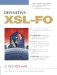B.2 XSL-FO functions

B.2.1 Function summary

Expressions can incorporate numbers and lengths as operands.

• A number does not include a unit of measure;

• this is termed "a unit power of zero."

• A length does include a unit of measure (inches, mm, etc.);

• this is termed "a unit power of one."

You can perform numeric operations on lengths.

• Some functions accept either lengths or numbers as operands, e.g.:

• font-size="min(10pt,1em)" ,

• <table- cell column-number="min(3,5)"> .

• Sometimes (e.g. for min() ), both operands must have the same unit power.

• Some functions do not work on lengths, only numbers.

• E.g. round() accepts only numbers, not lengths;

• to round a length, you need to convert it to a number, round, then convert the result back to a length, e.g.:

• round(.75in div 1in)*1in .

Percentages are counted in 1/100 units.

• They can be utilized in a property as a relation to the property's current value.

• The following expressions all evaluate to the same value:

• font-size="150%" ,

• font-size="1.5 * inherited-property-value(font-size)" ,

• font-size="1.5em" .

B.2.2 Function groupings

Color functions ( 5.10.2 )
• rgb() ,

• rgb-icc() ,

• system-color() .

Font functions ( 5.10.3 )
• system-font() .

• abs() ,

• ceiling() ,

• floor() ,

• max() ,

• min() ,

• round() .

Property value functions ( 5.10.4 )
• body-start() ,

• from- nearest -specified-value() ,

• from-parent() ,

• from-table-column() ,

• inherited-property-value() ,

• label-end() ,

• merge-property-values() ,

• proportional-column-width() .

B.2.3 Functions summarized by name

numeric abs( numeric ) ( 5.10.1 ):

• returns the absolute value of number or length argument.

numeric body-start() ( 5.10.4 ):

• returns the start indent corresponding to the list item body when considering the provisional-valued properties of the list.

numeric ceiling( numeric ) ( 5.10.1 ):

• returns the nearest integer closest to positive infinity.

numeric floor( numeric ) ( 5.10.1 ):

• returns the nearest integer closest to negative infinity.

object from-nearest-specified-value( NCName ) ( 5.10.4 ):

• returns the value of the named property from the nearest ancestor of the formatting object in which the property value is being explicitly specified,

• returns the initial value if there is no parent.

object from-parent( NCName ) ( 5.10.4 ):

• returns the value of the named property from the parent of the formatting object of the property being evaluated,

• returns the initial value if there is no parent.

object from-table-column( NCName ) ( 5.10.4 ):

• returns the value of the named property from the table-column corresponding to the current column in the table.

object inherited-property-value( NCName ) ( 5.10.4 ):

• returns the value of the inherited property named,

• reports an error if the property named is not an inherited property.

numeric label-end() ( 5.10.4 ):

• returns the end indent corresponding to the list item label when considering the provisional-valued properties of the list.

numeric max( numeric , numeric ) ( 5.10.1 ):

• returns the maximum of two arguments,

• allows the arguments to be either lengths or numbers, but they must be the same type.

object merge-property-values( NCName ) ( 5.10.4 ):

• returns the property calculated from the property set corresponding to the current user -agent state within the parent's child property sets.

numeric min( numeric , numeric ) ( 5.10.1 ):

• returns the minimum of two arguments,

• allows the arguments to be either lengths or numbers, but they must be the same type.

numeric proportional-column-width( numeric ) ( 5.10.4 ):

• returns the length corresponding to the number of units of proportional measure of the current table column's table as indicated in the supplied argument.

• Note: the proportional measure is the length left over after subtracting specified column widths from the table width and dividing the result by the number of columns for which widths are not specified.

color rgb( numeric , numeric , numeric ) ( 5.10.2 ):

• returns a color from the RGB space,

• allows arguments to be numbers, not lengths.

color rgb-icc( numeric , numeric , numeric , NCName , numeric , numeric ) ( 5.10.2 ):

• returns a color from the named ICC color profile,

• interprets the first three arguments as the fallback RGB color if the ICC color is not found,

• interprets the last arguments in a way specific to the color profile.

numeric round( numeric ) ( 5.10.1 ):

• returns an integer closest to the given number,

• returns ceiling() on the value .5 .

color system-color( NCName ) ( 5.10.2 ):

• returns the system-defined color named in the argument.

object system-font( NCName , NCName ) ( 5.10.3 ):

• returns the font size characteristic named in the second argument of the system font named in the first argument;

• if the second argument is omitted, the characteristic is the one being assigned by the expression.Definitive XSL-FO
ISBN: 0131403745
EAN: 2147483647
Year: 2002
Pages: 99
Authors: G. Ken Holman

Similar book on Amazon